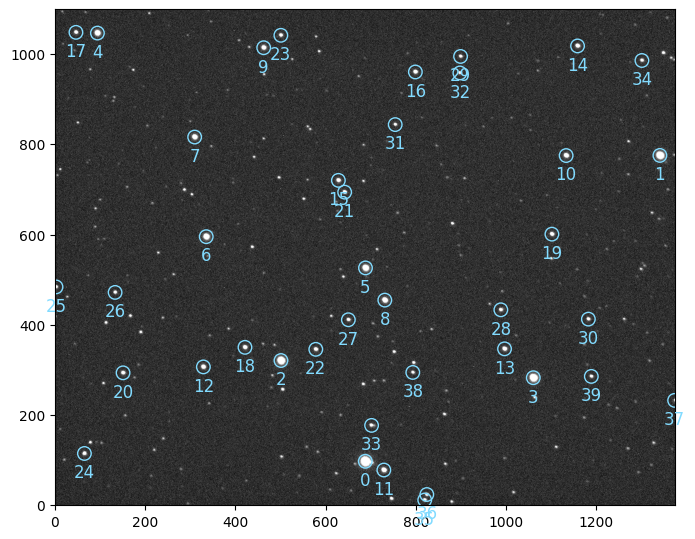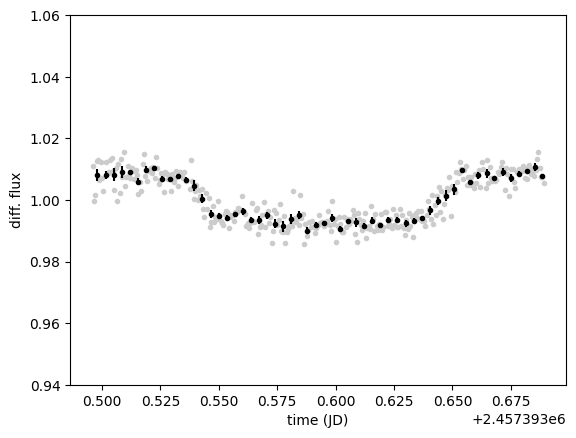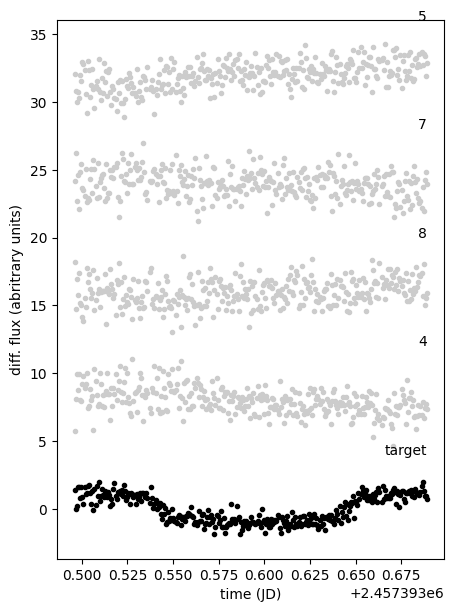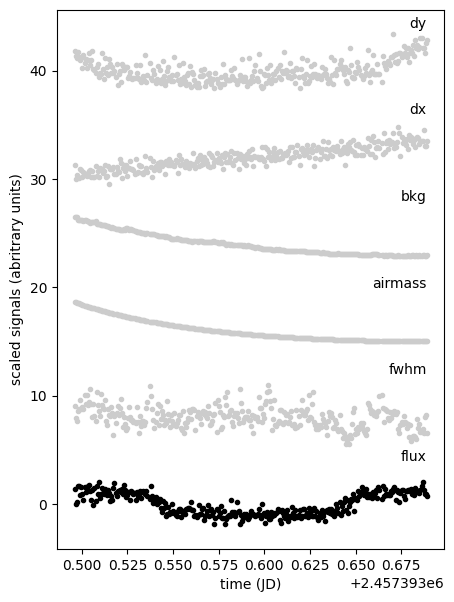# Exoplanet transit#

In this tutorial we will reduce raw images to produce a transit light curve of WAPS-12 b. All images can be downloaded from https://astrodennis.com/.

## Managing the FITS#

For this observation, the headers of the calibration images do not contain information about the nature of each image, bias, dark or flat. We will then retrieve our images by hand (where usually we would employ a FitsManager object)

from glob import glob
from pathlib import Path

folder = Path("/Users/lgarcia/data/wasp12_example_dataset")

darks = glob(str(folder / "Darks/*.fit"))
bias = glob(str(folder / "Bias/*.fit"))
flats = glob(str(folder / "Flats/*.fit"))
sciences = sorted(glob(str(folder / "ScienceImages/*.fit")))


## The full reduction#

What follows is the full reduction sequences, including the selection of a reference image to align sources and scale apertures on. More details are provided in the basic Photometry tutorial

import numpy as np
from prose import FITSImage, Sequence, blocks

# reference is middle image
ref = FITSImage(sciences[len(sciences) // 2])

calibration = Sequence(
[
blocks.Calibration(darks=darks, bias=bias, flats=flats),
blocks.Trim(),
blocks.PointSourceDetection(n=40),  # stars detection
blocks.Cutouts(21),  # stars cutouts
blocks.MedianEPSF(),  # building EPSF
blocks.psf.Moffat2D(),  # modeling EPSF
]
)

calibration.run(ref, show_progress=False)

photometry = Sequence(
[
calibration,  # calibration block (same as above)
blocks.PointSourceDetection(n=12, minor_length=8),  # fewer stars detection
blocks.Cutouts(21),  # stars cutouts
blocks.MedianEPSF(),  # building EPSF
blocks.Gaussian2D(ref),  # modeling EPSF with initial guess
blocks.ComputeTransformTwirl(ref),  # compute alignment
blocks.AlignReferenceSources(ref),  # alignment
blocks.AperturePhotometry(),  # aperture photometry
blocks.AnnulusBackground(),  # annulus background
blocks.GetFluxes(
"fwhm",
dx=lambda im: im.transform.translation,
dy=lambda im: im.transform.translation,
),
]
)

photometry.run(sciences)


Warning

For image alignment only n=12 sources are being detected, but note that these sources are not the ones for which the photometry is extracted. Indeed, the following block AlignReferenceSources(ref) modifies the sources from each image, setting them to the sources of the references but aligned to each image.

We can check the total processing time of each block with

photometry

╒═════════╤════════╤═══════════════════════╤════════════════╕
│   index │ name   │ type                  │ processing     │
╞═════════╪════════╪═══════════════════════╪════════════════╡
│       0 │        │ Calibration           │ 1.755 s (4%)   │
├─────────┼────────┼───────────────────────┼────────────────┤
│       1 │        │ PointSourceDetection  │ 18.141 s (41%) │
├─────────┼────────┼───────────────────────┼────────────────┤
│       2 │        │ Cutouts               │ 2.075 s (5%)   │
├─────────┼────────┼───────────────────────┼────────────────┤
│       3 │        │ MedianEPSF            │ 0.165 s (0%)   │
├─────────┼────────┼───────────────────────┼────────────────┤
│       4 │        │ Gaussian2D            │ 2.907 s (7%)   │
├─────────┼────────┼───────────────────────┼────────────────┤
│       5 │        │ ComputeTransformTwirl │ 0.494 s (1%)   │
├─────────┼────────┼───────────────────────┼────────────────┤
│       6 │        │ AlignReferenceSources │ 0.125 s (0%)   │
├─────────┼────────┼───────────────────────┼────────────────┤
│       7 │        │ CentroidQuadratic     │ 1.312 s (3%)   │
├─────────┼────────┼───────────────────────┼────────────────┤
│       8 │        │ AperturePhotometry    │ 12.040 s (27%) │
├─────────┼────────┼───────────────────────┼────────────────┤
│       9 │        │ AnnulusBackground     │ 5.607 s (13%)  │
├─────────┼────────┼───────────────────────┼────────────────┤
│      10 │        │ GetFluxes             │ 0.024 s (0%)   │
╘═════════╧════════╧═══════════════════════╧════════════════╛


## Differential photometry#

Now that the photometry has been extracted, let’s focus on our target and produce a differential light curve for it.

All fluxes have been saved in the GetFluxes block, in a Fluxes object

from prose import Fluxes

fluxes: Fluxes = photometry[-1].fluxes


We can check the reference image, on which all images sources have been aligned, and pick our target

ref.show()set this target (source 6) in the Fluxes object and proceed with automatic differential photometry

import matplotlib.pyplot as plt

fluxes.target = 6

# a bit of cleaning
nan_stars = np.any(np.isnan(fluxes.fluxes), axis=(0, 2)) # stars with nan fluxes
fluxes = fluxes.sigma_clipping_data(bkg=3, fwhm=3) # sigma clipping

# differential photometry
diff = fluxes.autodiff()

# plotting
ax = plt.subplot(xlabel="time (JD)", ylabel="diff. flux", ylim=(0.94, 1.06))
diff.plot()
diff.bin(5 / 60 / 24, estimate_error=True).errorbar()And here is our planetary transit. To validate the differential photometry and the automatic choice of comparison stars, we can plot their light curves along the target light curve

plt.figure(None, (5, 7))
ax = plt.subplot(xlabel="time (JD)", ylabel="diff. flux (abritrary units)")

# plotting only the first five comparisons
for j, i in enumerate([diff.target, *diff.comparisons[0:5]]):
y = diff.fluxes[diff.aperture, i].copy()
y = (y - np.mean(y)) / np.std(y) + 8 * j
plt.text(
diff.time.max(), np.mean(y) + 4, i if i != diff.target else "target", ha="right"
)
plt.plot(diff.time, y, ".", c="0.8" if i != diff.target else "k")# Explanatory measurements#

To help modeling the light curve, some explanatory measurements have been stored in

diff.dataframe

bkg airmass dx dy fwhm time flux
0 261.908217 1.878414 -0.314490 0.566460 4.823861 2.457393e+06 1.011125
1 263.309778 1.870291 -1.088033 0.466066 4.473168 2.457393e+06 0.999756
2 261.167119 1.862163 -0.868474 0.423316 4.369253 2.457393e+06 1.001618
3 252.933837 1.854302 -0.953985 0.551378 4.677914 2.457393e+06 1.012742
4 252.841623 1.846374 -1.025708 0.448884 4.735902 2.457393e+06 1.012824
... ... ... ... ... ... ... ...
327 157.205167 1.013443 1.007175 0.613441 4.130921 2.457394e+06 1.015685
328 157.248958 1.013356 1.577194 0.623359 4.012928 2.457394e+06 1.010365
329 156.650647 1.013278 0.737424 0.519273 4.491135 2.457394e+06 1.007430
330 156.423297 1.013210 0.700936 0.711975 4.576204 2.457394e+06 1.007304
331 157.131704 1.013150 0.977755 0.784270 4.015622 2.457394e+06 1.005600

332 rows × 7 columns

that we can plot to check for any correlation with the differential flux

plt.figure(None, (5, 7))
ax = plt.subplot(xlabel="time (JD)", ylabel="scaled signals (abritrary units)")

for i, name in enumerate(["flux", "fwhm", "airmass", "bkg", "dx", "dy"]):
y = diff.df[name].copy()
y = (y - np.mean(y)) / np.std(y) + 8 * i
plt.text(diff.time.max(), np.mean(y) + 4, name, ha="right")
plt.plot(diff.time, y, ".", c="0.8" if name != "flux" else "k")## Multiprocessing alternative#

For those in a hurry, the photometry sequence can be made parallel

from prose.core.sequence import SequenceParallel

faster_photometry = SequenceParallel(
[
blocks.Calibration(darks=darks, bias=bias, flats=flats, shared=True),
blocks.PointSourceDetection(
n=12, minor_length=8
),  # stars detection for alignment
blocks.Cutouts(21),  # stars cutouts
blocks.MedianEPSF(),  # building EPSF
blocks.Gaussian2D(ref),  # modeling EPSF with initial guess
blocks.ComputeTransformTwirl(ref),  # compute alignment
blocks.AlignReferenceSources(ref),  # align sources
blocks.AperturePhotometry(),  # aperture photometry
blocks.AnnulusBackground(),  # annulus background
blocks.Del("data", "sources", "cutouts"),  # (optional) reduce overhead
],
[
blocks.GetFluxes(
"fwhm",